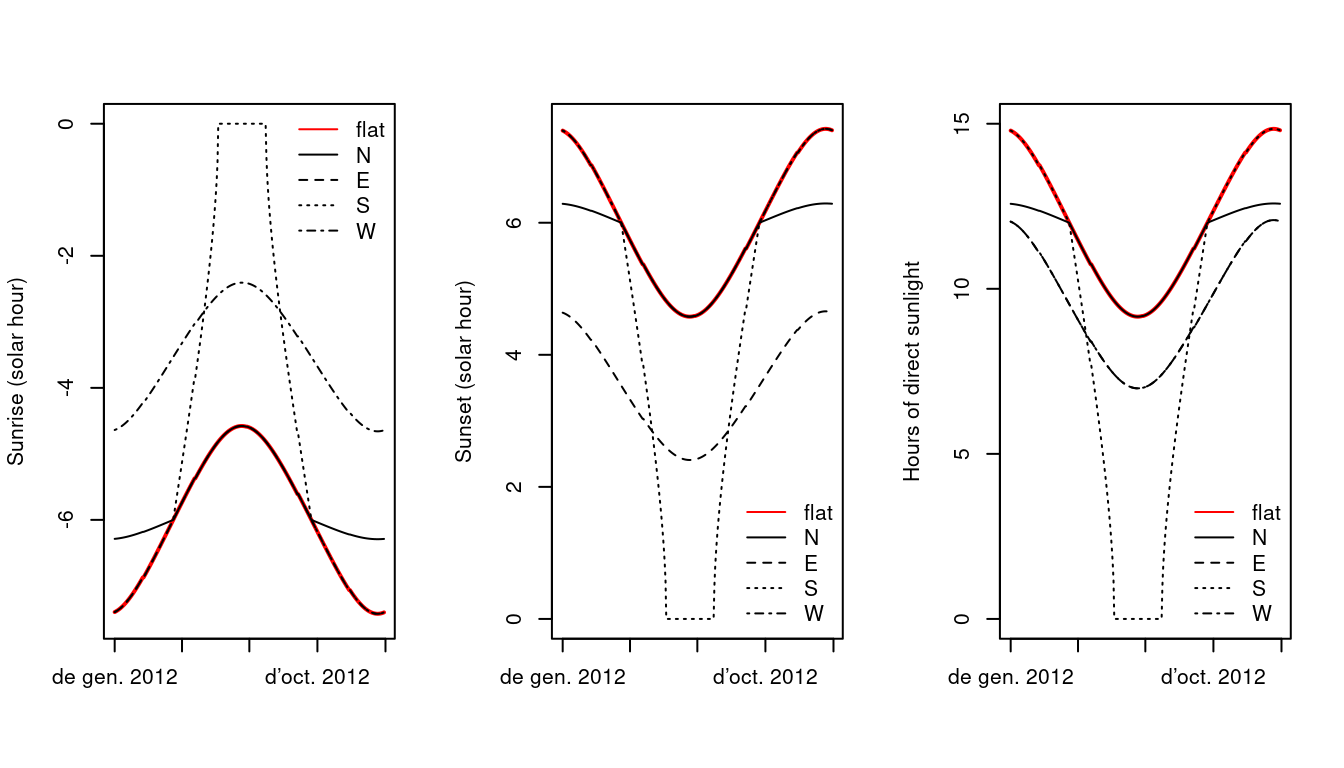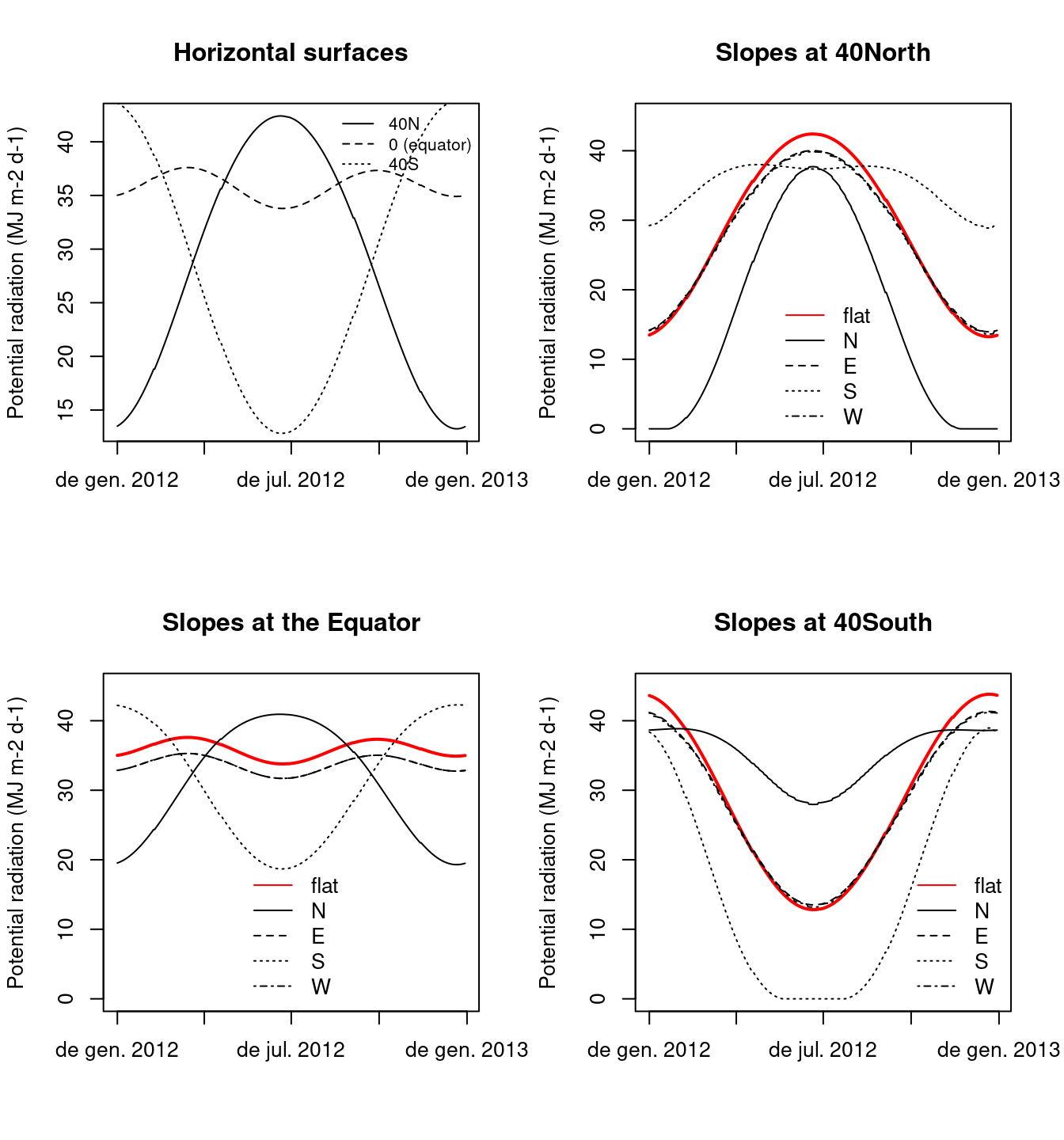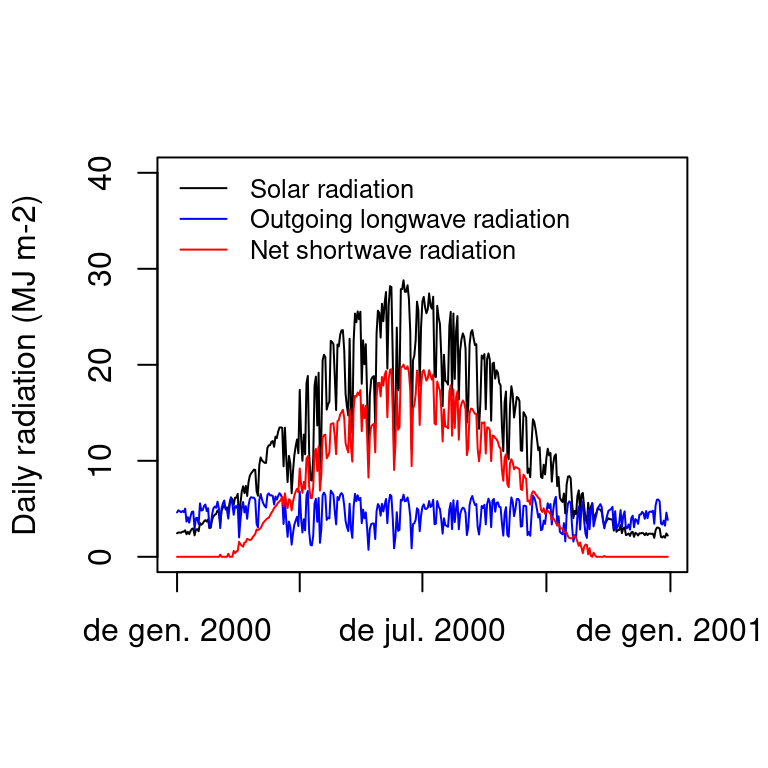# Chapter 5 Statistical correction of weather data

Statistical correction is necessary when meteorological data is available at a spatial scale that is too coarse for landscape-level analysis. This is usually the case when taking predictions from global or regional climate models. The general idea of correction to the landscape level is that a fine-scale meteorological series is to be compared to coarse-scale series for the a historical (reference) period. The result of this comparison can be used to correct coarse-scale meteorological series for other periods (normally future projections).

## 5.1 Correction methods

Users of meteoland can choose between three different types of corrections:

• Unbiasing: consists in subtracting, from the series to be corrected, the average difference between the two series for the reference period . Let $$x_i$$ be the value of the variable of the more accurate (e.g. local) series for a given day $$i$$ and $$u_i$$ the corresponding value for the less accurate series (e.g., climate model output). The bias, $$\theta$$, is the average difference over all $$n$$ days of the reference period: $\begin{equation} \theta = \sum_{i}^{n}(u_i - x_i)/n \end{equation}$ The bias calculated in the reference period is then subtracted from the value of $$u$$ for any day of the period of interest.
• Scaling: A slope is calculated by regressing $$u$$ on $$x$$ through the origin (i.e. zero intercept) using data of the reference period. The slope can then be used as scaling factor to multiply the values of $$u$$ for any day of the period of interest.
• Empirical quantile mapping: Due to its distributional properties, neither multiplicative or additive factors are appropriate for daily precipitation . In this case, it has been recommended to compare the empirical cumulative distribution function (CDF) of the two series for the reference period . The empirical CDFs of $$x$$ and $$u$$ for the reference period are approximated using tables of empirical percentiles, and this mapping is used to correct values of $$u$$ for the period of interest: $\begin{equation} c_d = ecdf_x^{-1}(ecdf_u(u_d)) \end{equation}$ where $$ecdf_x$$ and $$ecdf_u$$ are the empirical CDFs of $$x$$ and $$u$$ respectively. Values between percentiles are approximated using linear interpolation. A difficulty arises for quantile mapping when the variables bounded by zero, such as precipitation. As the models tend to drizzle (or may have lower frequency of precipitation events), the probability of precipitation in the model may be greater or lower than that observed. To correct this, when model precipitation is zero an observed value is randomly chosen in the interval where the observed cumulative frequency is less than or equal to the probability of no precipitation in the model. This procedure ensures that the probability of precipitation after correction is equal to that observed .

For each target location to be processed, the correction routine first determines which is the nearest climate model cell and extracts its weather data series for the reference period and the period of interest. Then, the correction method chosen by the user for each variable is applied. Statistical corrections are done for each of the twelve months separately to account for seasonal variation of distributional differences .

## 5.2 Default approaches by variable

Although users can choose their preferred correction method for each variable, meteoland has default approaches.

Precipitation

By default, correction of precipitation is done using empirical quantile mapping. The following figures show the correction of RCM precipitation predictions for 2023 using interpolated data from 2002-2003 as observations for the reference period. Note that at least 15 years of observations (and not two!) would be needed for a correct estimation of monthly CDFs.Mean temperature

Unbiasing method is used by default to correct mean temperature. The following figures show the correction of RCM mean temperature predictions for 2023 using interpolated data from 2002-2003 as observations for the reference period:Minimum and maximum temperatures

To correct minimum (respectively maximum) temperature values, by default scaling is applied to the difference between minimum (resp. maximum) temperature and mean temperature.Radiation is by default corrected using the unbiasing procedure. The following figures show the correction of RCM radiation predictions for 2023 using interpolated data from 2002-2003 as observations for the reference period:Relative humidity

Mean relative humidity is first transformed to specific humidity, the unbiasing method is applied by default to this variable and the result is back transformed to mean, minimum and maximum relative humidity using the previously corrected series of mean, maximum and minimum temperature, respectively.

Wind speed

By default, wind speed is corrected using the scaling method. Since historic wind data is often not available, however, if wind speed data is missing the coarse-scale wind estimate is taken directly without correction.

### Bibliography

Boé, J, L Terray, F Habets, and E Martin. 2007. Statistical and dynamical downscaling of the Seine basin climate for hydro-meteorological studies.” International Journal of Climatology 27: 1643–55. https://doi.org/10.1002/joc.1602.
Déqué, Michel. 2007. Frequency of precipitation and temperature extremes over France in an anthropogenic scenario: Model results and statistical correction according to observed values.” Global and Planetary Change 57 (1-2): 16–26. https://doi.org/10.1016/j.gloplacha.2006.11.030.
Gudmundsson, L., J. B. Bremnes, J. E. Haugen, and T. Engen-Skaugen. 2012. Technical Note: Downscaling RCM precipitation to the station scale using statistical transformations - A comparison of methods.” Hydrology and Earth System Sciences 16 (9): 3383–90. https://doi.org/10.5194/hess-16-3383-2012.
Ruffault, Julien, Nicolas K Martin-StPaul, Carole Duffet, Fabien Goge, and Florent Mouillot. 2014. Projecting future drought in Mediterranean forests: bias correction of climate models matters! Theoretical and Applied Climatology 117 (1-2): 113–22. https://doi.org/10.1007/s00704-013-0992-z.